# Differential equations and numerical methods questions

i have few doubts about differential equations and numerical methods ...
in a differential equation question ... you are given an instantaneous rate of change...
and you are supposed to find the function that gives , this instantaneous rate of change

Numerical methods approximate the "instantaneous rate of change" with "the rate of change over a small interval".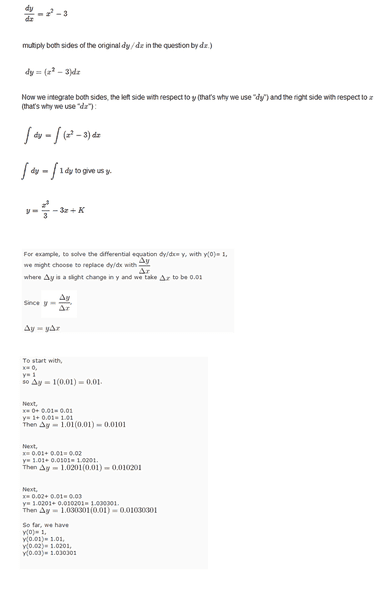is this same as ...

f(0)=1,
f(0.01)=1.01,
f(0.02)=1.0201,
f(0.03)= 1.030301

??

BvU
Homework Helper
Yes. Note that replacing ##\ dy\over dx\ ## by ##\ \Delta y\over \Delta x\ ## is an approximation....

thanks ...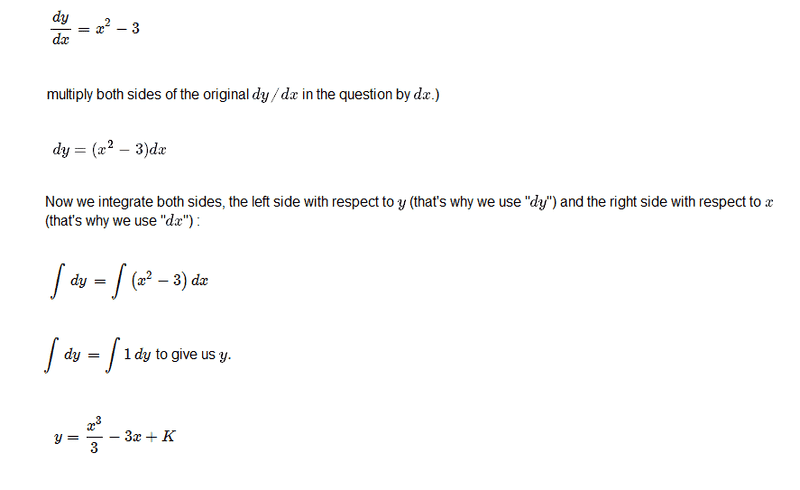does anyone know how to re write this equation for numerical approximations ??

BvU
Homework Helper
No approximation needed: you have solved the equation....

i am trying to understand some things in plain english ...
in a differential equation question,you are given an instantaneous rate of change of an object
The gradient of the curve dy/dx is the instantaneous change of y with respect to x ,the rate at which y is changing as x is changing
and you are supposed to find the function that gives , this instantaneous rate of changeand this is same as ...

f(0)=1,
f(0.01)=1.01,
f(0.02)=1.0201,
f(0.03)= 1.030301

??

wasn't i supposed to find the function that gives ,this instantaneous rate of change ??
The answer is the function that has that rate of change
The answer is a function that has the instantaneous rate of change that you were given ...

now i have four functions and four instantaneous rate of change ??
what does this mean ??
does it mean that i have four functions that has the instantaneous rate of change that i was given

??

BvU
Homework Helper
You have two equations with a different character: one (##{dy\over dx}=x^2-3##) is of the type ## y'= f(x) ## and the other is of the type ##y'= f(y)## (I use the abbreviation ##y'## for the derivative ##{dy\over dx}## ).

## y'= f(x) ## is solved if you can find a primitive of f : a function ##F(x)## for which ##F'= f##.

There is the small matter of the integration constant: If you know your rate of change but you don't know where you start, then you can not determine where you will be next.

In other words: With every differential eqiuation you need something to deal with the integration constant (one for every "quote") . For example a function value at x = 0 (or a function value at any other point).

Your first equation isn't accompanied by such a condition, so you can't get further than ## y = F(x) = x^3/3-3x + K##.

Your second differential equation does have a condition: ##y(0) = 1##. You solve numerically by approaching y(0) as a linear function for a small step:$$y(0+\Delta x) \approx y(0) + \Delta x {dy\over dx}$$ when all you really know is $$\lim_{h\downarrow 0} { y(0+h) - y(0) \over h } = {dy\over dx} \ .$$
When you take a few steps you get a sequence of approximated function values, but not a function (in the sense of an exact prescription what to do with x to get y).

So for ##\ y'= y\ \ \& \ \ y(0) = 1 \ ## you have found four function values, not four functions.

Last edited:
You have two equations with a different character: one (##{dy\over dx}=x^2-3##) is of the type ## y'= f(x) ## and the other is of the type ##y'= f(y)## (I use the abbreviation ##y'## for the derivative ##{dy\over dx}## ).

## y'= f(x) ## is solved if you can find a primitive of f : a function ##F(x)## for which ##F'= f##.

There is the small matter of the integration constant: If you know your rate of change but you don't know where you start, then you can not determine where you will be next.

In other words: With every differential eqiuation you need something to deal with the integration constant (one for every "quote") . For example a function value at x = 0 (or a function value at any other point).

Your first equation isn't accompanied by such a condition, so you can't get further than ## y = F(x) = x^3/3-3x + K##.

Your second differential equation does have a condition: ##y(0) = 1##. You solve numerically by approaching y(0) as a linear function for a small step:$$y(0+\Delta x) \approx y(0) + \Delta x {dy\over dx}$$ when all you really know is $$\lim_{h\downarrow 0} { y(0+h) - y(0) \over h } = {dy\over dx} \ .$$
When you take a few steps you get a sequence of approximated function values, but not a function (in the sense of an exact prescription what to do with x to get y).

So for ##y'= y & y(0) = 1 ## you have found four function values, not four functions.

BvU ,

thanks a lot .. there is so much good information in that post ... i have already learned a lot from your post ... now i think its just a matter of googling the proper words from your post ..

even differential equation is kind of getting interesting ...

few more basic questions ...

i dont understand this properly ...

most of the time , the original question is ...

find the function that gives ,this instantaneous rate of change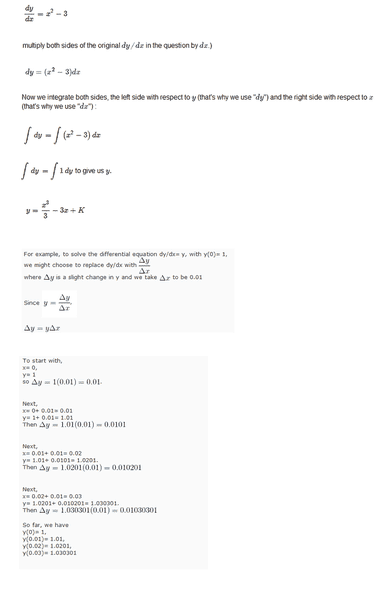which is same as this ..

f(0)=1,
f(0.01)=1.01,
f(0.02)=1.0201,
f(0.03)= 1.030301

for ##y'= y & y(0) = 1 ## you have found four function values, not four functions.

what is the next thing you do with these function values... you plug it into the equations ??

i really thought the answer is a function ..that has that rate of change

i mean this is how a question really starts ...

find the function that gives ,this instantaneous rate of change

<Mod note: Irrelevant image deleted>

if answer is a function that has that rate of change ...

what are these four function values ...

???

Last edited by a moderator:
BvU
Homework Helper
Nice picture; taken from inside the book ? (I recognize Rotterdam, though...)

Seems to me you still have trouble understanding what you did for ##y'=y## so let's step back and treat ##y'= x^2-3## the same way. Add initial condition y(0) = 0 so it yields a unique solution.

##x = 0 \rightarrow y(0) = 0##

##x = 0.1 \rightarrow y(0.1) = 0 + (0.01 - 3)* 0.1 = -0.299 \ ## exact solution ##y(0.1) = 0.001 - 3 = -0.299 \ ## hurray.

etc. etc. we get: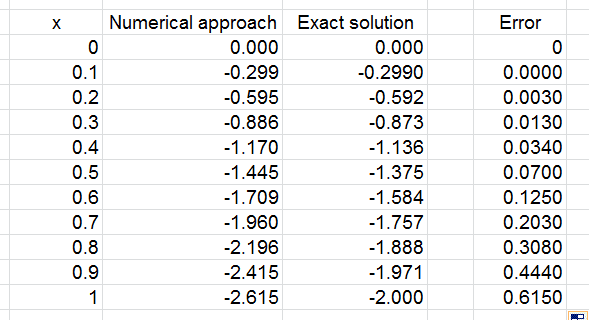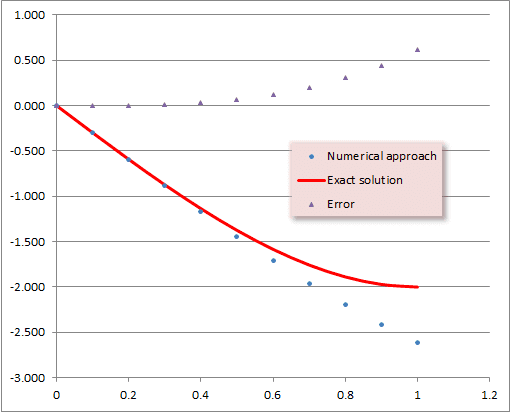So the exact solution is a function ##y = x^3/3-3x## and the numerical approach is a table of aproximated function values.

(I took steps of 0.1 so the difference between exact and numerical becomes visible).

In fact, for your other equation ##y'=y## and ##y(0)=1## an exact solution can also be found. Use the same method (everything with y on one side gives ##{dy\over y}=dx##, then integrate and apply the initial condition). But you'll learn that one soon enough.

#### Attachments

let me re arrange a few things ...so that i can understand this properly ...

ok , there are two questions in this one ...

find the function that gives, this instantaneous rate of change ...which is same as this ..

f(0)=1,
f(0.01)=1.01,
f(0.02)=1.0201,
f(0.03)= 1.030301

You have two equations with a different character: one (##{dy\over dx}=x^2-3##) is of the type ## y'= f(x) ## and the other is of the type ##y'= f(y)## (I use the abbreviation ##y'## for the derivative ##{dy\over dx}## ).

## y'= f(x) ## is solved if you can find a primitive of f : a function ##F(x)## for which ##F'= f##.

There is the small matter of the integration constant: If you know your rate of change but you don't know where you start, then you can not determine where you will be next.

In other words: With every differential eqiuation you need something to deal with the integration constant (one for every "quote") . For example a function value at x = 0 (or a function value at any other point).

Your first equation isn't accompanied by such a condition, so you can't get further than ## y = F(x) = x^3/3-3x + K##.

Your second differential equation does have a condition: ##y(0) = 1##. You solve numerically by approaching y(0) as a linear function for a small step:$$y(0+\Delta x) \approx y(0) + \Delta x {dy\over dx}$$ when all you really know is $$\lim_{h\downarrow 0} { y(0+h) - y(0) \over h } = {dy\over dx} \ .$$
When you take a few steps you get a sequence of approximated function values, but not a function (in the sense of an exact prescription what to do with x to get y).

So for ##\ y'= y\ \ \& \ \ y(0) = 1 \ ## you have found four function values, not four functions.

for ##y'= y & y(0) = 1 ## you have found four function values, not four functions.

for ##f(x)=y & f(0) = 1 ## you have found four function values, not four functions.

f(0)=1,
f(0.01)=1.01,
f(0.02)=1.0201,
f(0.03)= 1.030301

Seems to me you still have trouble understanding what you did for ##y'=y## so let's step back and treat ##y'= x^2-3## the same way. Add initial condition y(0) = 0 so it yields a unique solution.

##x = 0 \rightarrow y(0) = 0##

##x = 0.1 \rightarrow y(0.1) = 0 + (0.01 - 3)* 0.1 = -0.299 \ ## exact solution ##y(0.1) = 0.001 - 3 = -0.299 \ ## hurray.

etc. etc. we get:
View attachment 103655

View attachment 103661

So the exact solution is a function ##y = x^3/3-3x## and the numerical approach is a table of aproximated function values.

(I took steps of 0.1 so the difference between exact and numerical becomes visible).

In fact, for your other equation ##y'=y## and ##y(0)=1## an exact solution can also be found. Use the same method (everything with y on one side gives ##{dy\over y}=dx##, then integrate and apply the initial condition). But you'll learn that one soon enough.

BvU ,

its from a book called "numerical methods for ordinary differential equations " ...i did a horizontal flip of that image with ms paint ..
Mod note: Why? How does that add any information to your question? Also, you have posted an image of a bridge twice, which also adds nothing of substance to what you're asking.
few more re arrangements in a way that i can understand this ...

Seems to me you still have trouble understanding what you did for ##f(x)=y## so let's step back and treat ##f(x)= x^2-3## the same way. Add initial condition f(0) = 0 so it yields a unique solution.

##x = 0 \rightarrow f(0) = 0##

##x = 0.1 \rightarrow f(0.1) = 0 + (0.01 - 3)* 0.1 = -0.299 \ ## exact solution ##f(0.1) = 0.001 - 3 = -0.299 \ ##

etc .. etc .. we get ..So the exact solution is a function ##f(x) = x^3/3-3x## and the numerical approach is a table of aproximated function values.

(I took steps of 0.1 so the difference between exact and numerical becomes visible).

In fact, for your other equation ##f(x)=y## and ##f(0)=1## an exact solution can also be found. Use the same method (everything with y on one side gives ##{dy\over y}=dx##, then integrate and apply the initial condition). But you'll learn that one soon enough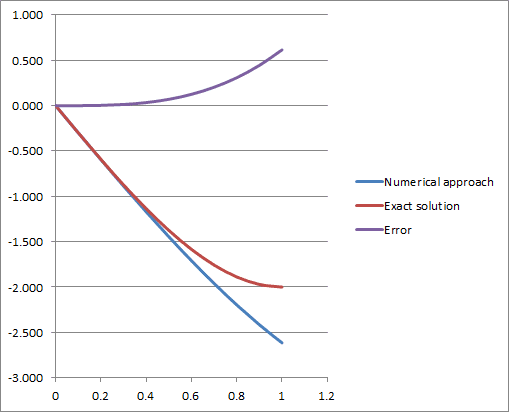i am going to take a little bit of time to read and re read this ...

there is so much good information again on this one ...

let me see what i can learn from this , and i don't know where to move to from here ...

again , thanks a lot ...

if i have more doubts i will come back and post more ...

Last edited by a moderator: